29

## 到死都没能飞起来23

## 引力势能和电势能@qiusir:榴莲似乎可以用来形容一种人，粗硬多刺的外表却裹着柔软丰实的心。不少人被其外表所拒，而能触及其内在的也未必受得那气味，但对敢能享食者来说却多有水果之王之美誉.
@qiusir:有的知识也如榴莲，不畏其表不惧味，能敢食之方知其美味.1、引力势能
$\int\frac{1}{r^2}dr=-\frac{1}{r}$
$E_p=-\int F\cdot dx$
$E_p(r)=-\int_{\infty }^{r}-\frac{GMm}{r^2}dr$
$E_p(r)=-\frac{GMm}{r}$
2、重力势能

$E_p=-\frac{GMm}{h+R}-(-\frac{GMm}{R})$
$E_p=\frac{GMm}{R}-\frac{GMm}{R+h}$
$E_p=\frac{GMm}{R}(1-\frac{1}{1+\frac{h}{R} })$

$E_p\approx\frac{GMm}{R^2}h$

3、电势能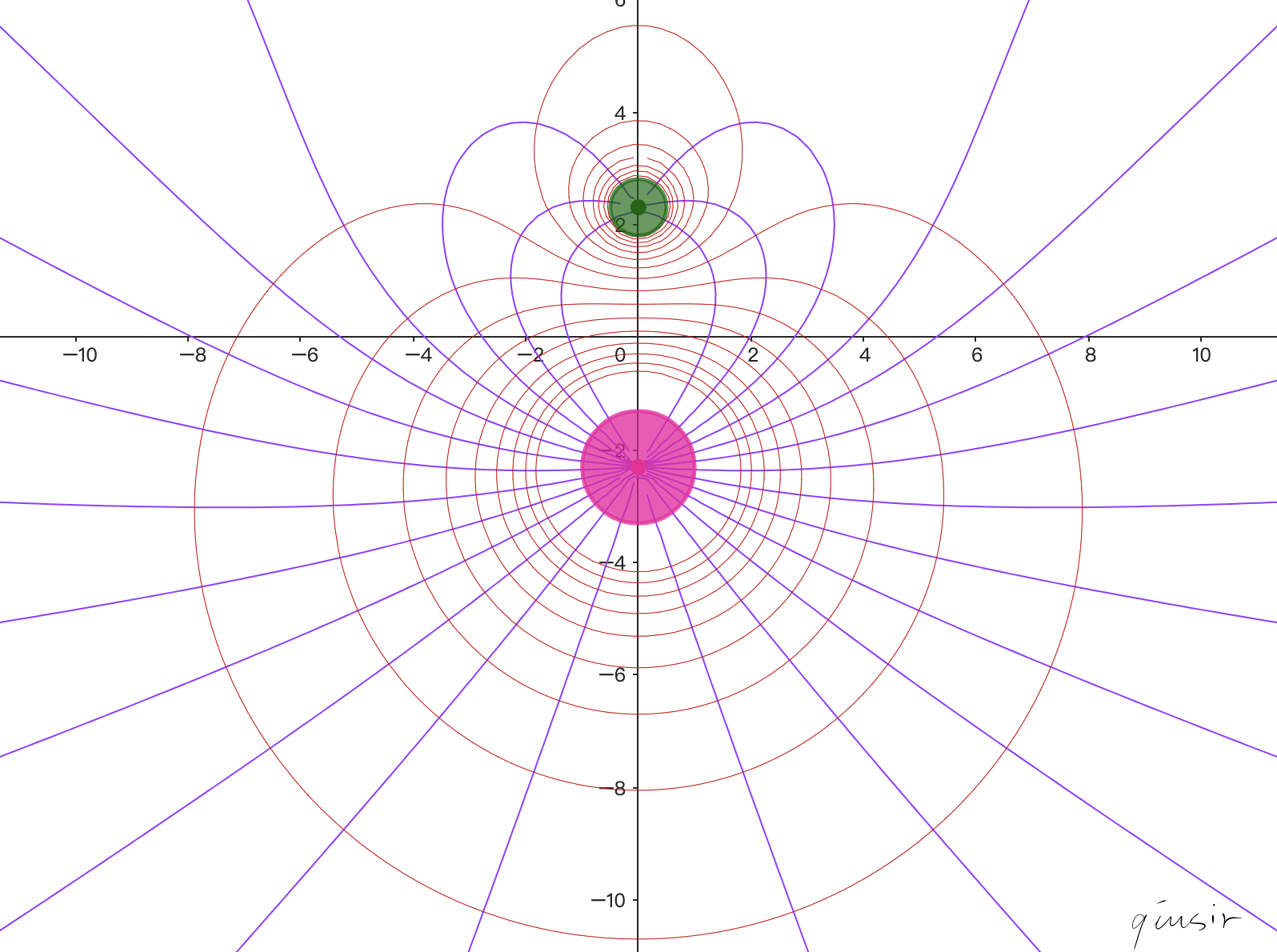$\varphi_n=\frac{k_0Q_1}{\sqrt{(x+l)^2+y^2}}+\frac{k_0Q_2}{\sqrt{(x-l)^2+y^2}}$

$\psi_n=\frac{k_0Q_1(x+l)}{\sqrt{(x+l)^2+y^2}}+\frac{k_0Q_2(x-l)}{\sqrt{(x-l)^2+y^2}}$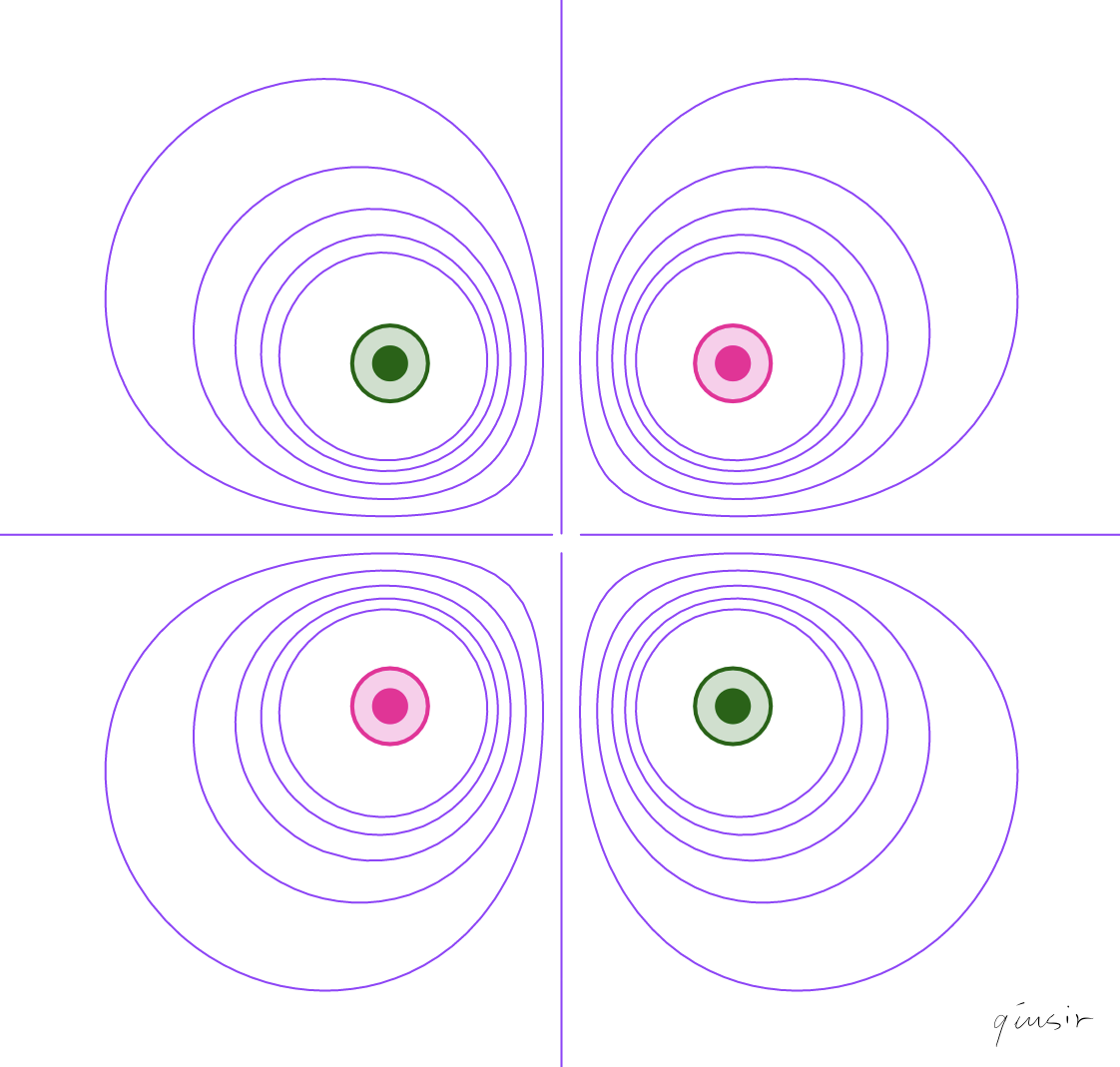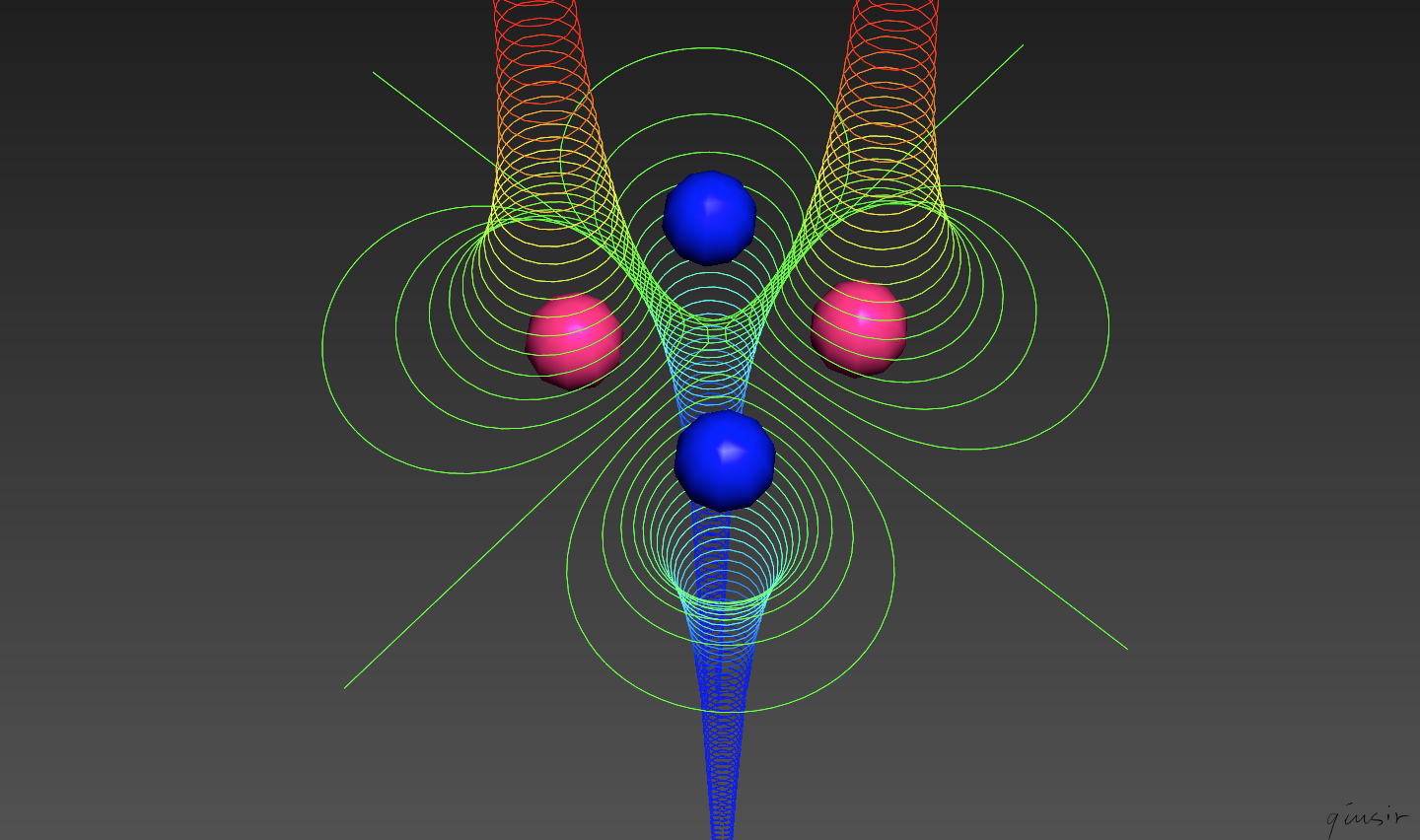22

## 我想跟自己谈谈金钱哲学

How to Worry Less About Money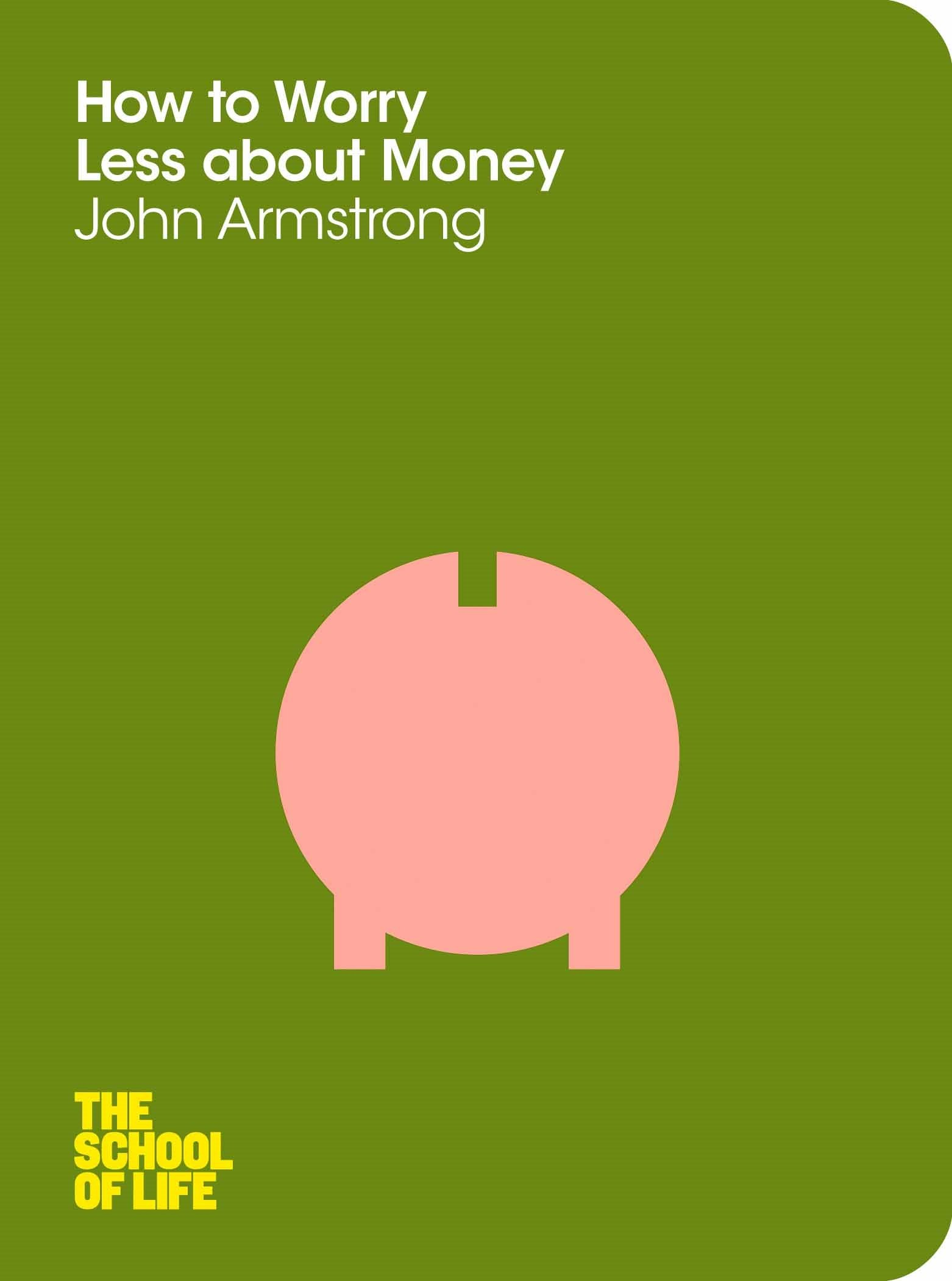1、引言

2、思考金钱
（过于谦逊，讨好型人格...）

3、金钱的神秘意义

4、金钱与美好生活

5、确定顺序

6、如何既赚钱同时又做好人

7、我们在社会中的位置

“我担心自己嫉妒得快吐了。”

...他觉得自己不理会自己的理智判断而忽视两人的社会阶层差异，己经算是对她莫大恩惠了。伊丽莎白通过看清达西先生的缺陷，聪明地保持着自己的尊严。

“他要求他用嘴巴叼起那枚金币，放到驴粪上。”

19

## 邴楚茗:浅谈约化质量与恢复系数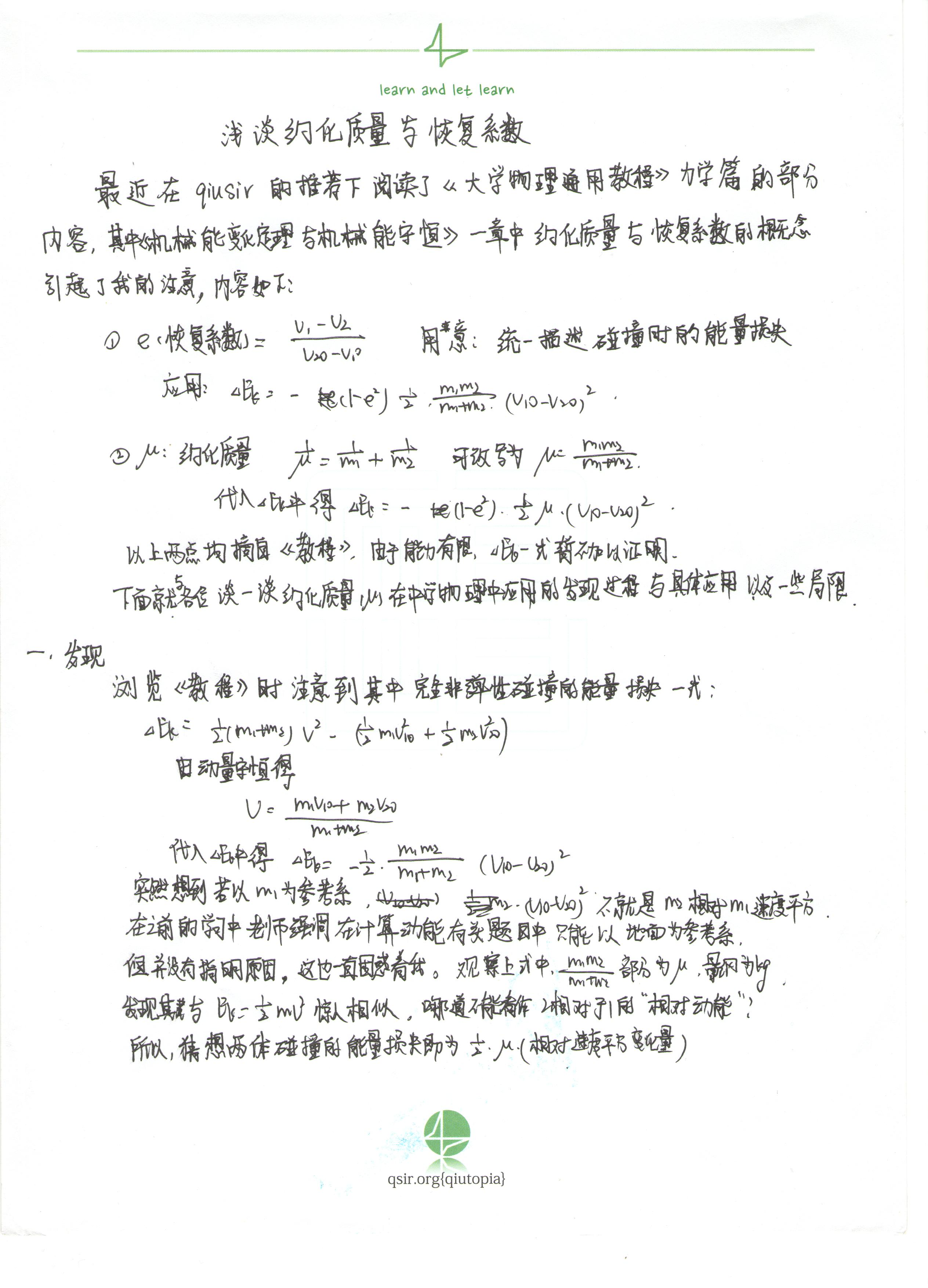17

## “幸福是自己的事”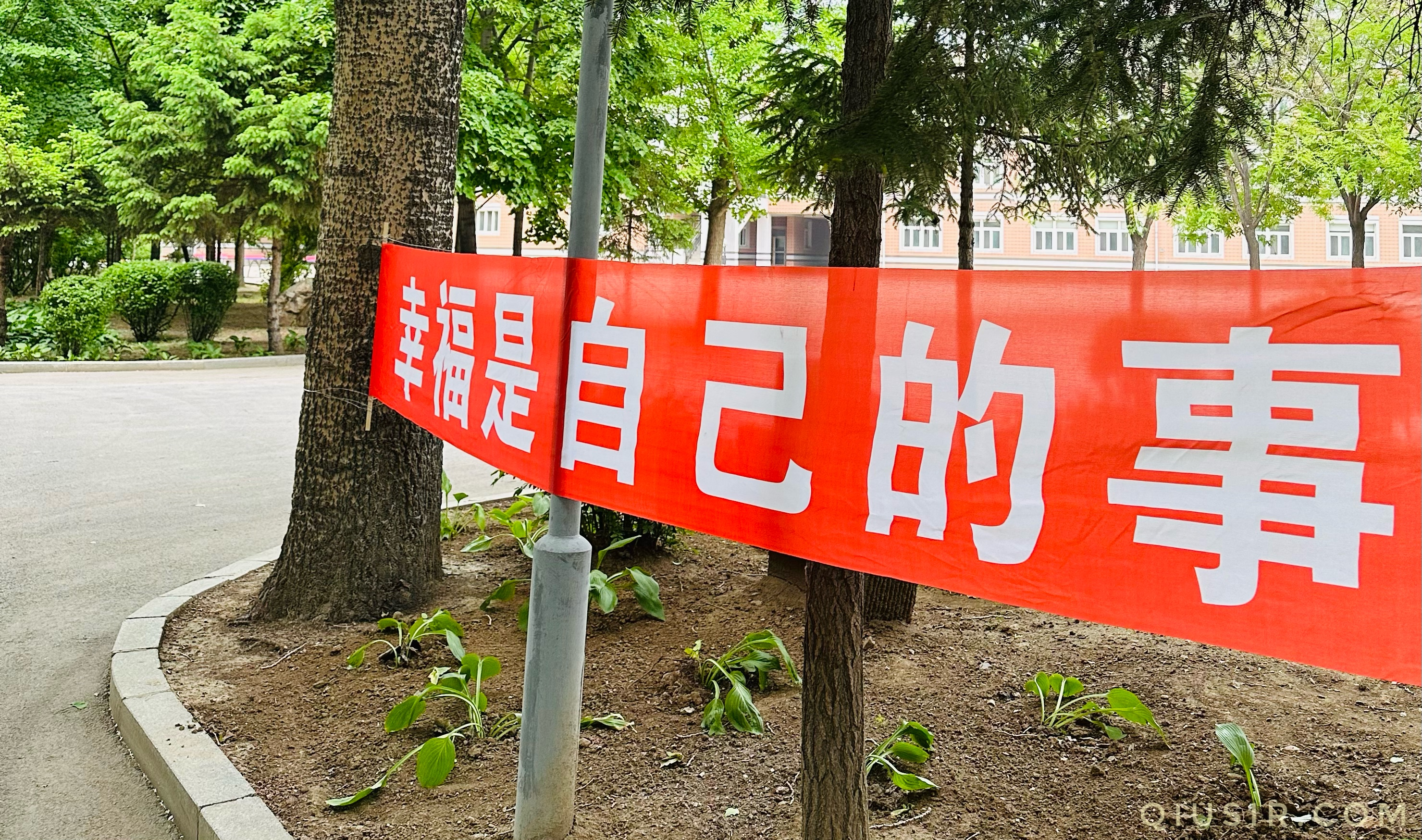Copilot如何评价“幸福是你自己的事”：

"幸福是自己的事"这样的话意味着每个人的幸福取决于自己的行为、态度和选择。它强调个体在追求幸福时应该主动承担责任，而不是依赖外部因素或他人的影响。这种观点鼓励人们独立思考、自我反省和积极行动，以实现他们自己定义的幸福。然而，要注意到人们的幸福受到社会、文化和环境等外部因素的影响，而且并非所有的幸福困境都可以完全由个人掌控。因此，在评估这样的话时，需要平衡个人责任和外部影响之间的关系，以建立一个综合的幸福理念

Copilot是如何评价“求师得”呢:)